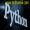# python函数坑之修改可变类型全局变量老董2020-09-29175围观,121赞

前言：函数的坑可以查看此文python函数各种坑了解更多。对于可变类型全局变量，在函数内部不需要global就可以直接修改(比如追加元素、删除元素、修改元素)，但是如果是在函数内部加global关键字也无妨。看下面两个案例：

```# -*- coding: utf-8 -*-

number = [1,2,3,4]

def change():
number.append('aaa')
print('函数内部',number,id(number))

change()
print('函数外部',number,id(number))

```
```函数内部 [1, 2, 3, 4, 'aaa'] 2630696734408

```

加global也没事，正常运行（有些朋友会误认为不管什么数据类型都必须加global才可以）。

```# -*- coding: utf-8 -*-

number = [1,2,3,4]
def change():
global number
number = 'aaa'
print('函数内部',number,id(number))

change()
print('函数外部',number,id(number))

def change():
global number
number.append('bbb')
print('函数内部',number,id(number))

change()
print('函数外部',number,id(number))
```
```函数内部 [1, 2, 'aaa', 4] 1369159077576

```

python编程网提示：转载请注明来源www.python66.com。

## 文章评论

python函数坑之修改可变类型全局变量文章写得不错，值得赞赏

## 站点信息

• 网站程序：Laravel
• 客服微信：a772483200